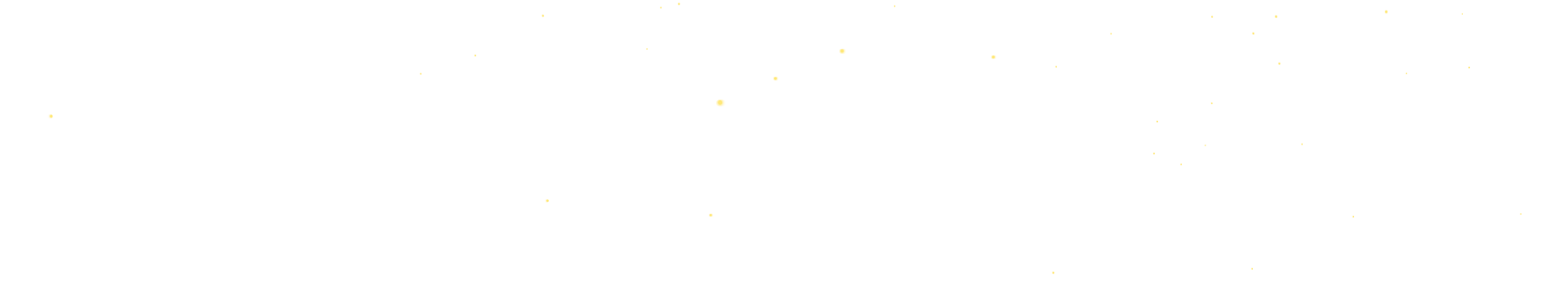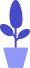# Animating with Python Pygame

Estimated reading: 10 minutes 49 views

We have learned to draw graphics with Python Pygame functions before, this section will learn to animate with Python Pygame

## Python Pygame load image

In addition to drawing various shapes on the screen ourselves, we can also load pictures in the program. In Pygame, the easiest way to manipulate an image is to call the image() function.

Let’s try loading a picture of a puppy in the Pygame window. First, copy the photos you want to load to the same location where you saved the Python program. In this way, Python can easily find this file when the program is running, without specifying the path where the image is stored. code show as below.

``````import pygame
pygame.init()
windowSurface = pygame.display.set_mode([800,600])
windowSurface.blit(pic, (100,200))
Running=True
while Running:
for event in pygame.event.get():
if event.type == pygame.QUIT:
Running=False
pygame.display.update()
pygame.quit()``````

Only the highlighted two lines of code in this code are newly added, the rest of the code we have seen before. The pygame.image.load() function loads an image from the hard disk, the name of the image file is “dog1.png”, and creates a Surface named pic. Then we call the blit() function to copy the pixels from one Surface to the other.

windowSurface.blit(pic, (100,100)) is to copy the pic object to the surface of screen. In this example, we tell blit() that we want to draw the pic to the position (100,200), which is the top left corner of the screen 100 pixels to the right and 200 pixels down.

Here we need to use the blit() function because the pygame.image.load() function works differently than the previous draw functions. All pygame.draw functions take a Surface as an argument, so by passing screen to pygame.draw.line() we can have pygame.draw.line() draw to the display window.

However, the pygame.image.load() function does not accept a Surface as an argument, instead it automatically creates a new Surface for the image. The image does not appear on the initial drawing screen unless blit() is used.

Run this program and get the display result shown in Figure 1.figure 1

## Python Pygame moving pictures

In addition to loading the puppy picture, we can also make the puppy move by changing the position of the picture. We can update the coordinates of the image in the game loop, and then draw the image at the new position each time the loop executes, so that it looks like the puppy is moving. code show as below.

``````import pygame
pygame.init()
windowSurface = pygame.display.set_mode([800,600])
picX = 0
picY = 200
Running=True
while Running:
for event in pygame.event.get():
if event.type == pygame.QUIT:
Running=False
picX += 1
windowSurface.blit(pic, (picX,picY))
pygame.display.update()
pygame.quit()``````

Among them, the changed code is still highlighted. Here, add two variables, picX and picY, that represent the X and Y coordinates of the image on the screen. Then in the while loop, increment the picX variable by 1 each time, so that the image can be shifted to the right.

As we mentioned earlier, the += operator is equivalent to adding 1 to the variable on the left. We also put the blit() function into the while loop, and use picX and picY to represent the X coordinate and the Y coordinate respectively, so that the displayed image can be updated every time.

Run the code to see the effect, as shown in Figure 2.figure 2
We can see that the puppy will keep moving to the right until it leaves the window, and it also leaves a trail of pixels on the window. Some pixels that deviate out.

If you want to fix this, you can fill the window to be drawn by calling the windowSurface.fill() function each time before copying the image. For example, it can be filled with black. Still the same, highlighted code means new code. The complete code is as follows.

``````import pygame
pygame.init()
windowSurface = pygame.display.set_mode([800,600])
picX = 0
picY = 200
BLACK=(0,0,0)
Running=True
while Running:
for event in pygame.event.get():
if event.type == pygame.QUIT:
Running=False
windowSurface.fill(BLACK)
picX += 1
windowSurface.blit(pic, (picX,picY))
pygame.display.update()
pygame.quit()``````

We define a constant called BLACK and assign it the tuple (0, 0, 0), which represents black. Then, a sentence windowSurface.fill(BLACK) is added to the while loop, indicating that the window must be refilled every time the loop is looped.

Let’s take a look at the execution effect, as shown in Figure 3.image 3
This time there is no trail of pixels left behind the moving image. By filling the window with black pixels, each frame of the old image in the window is erased, and then a new image is drawn in the new position. This achieves the effect of smooth movement, as shown in Figure 4.Figure 4

## Impact checking

Now there is a new problem, that is, the puppy will soon run out of the screen. In order to make the program more interesting, we can set a boundary for the screen. When it is detected that the puppy touches this boundary, it will change its movement. direction, let it turn around and run back. The concept of collision detection will be used here.

Collision detection is responsible for checking and handling the situation where two objects on the computing screen come into contact with each other (ie, collide). For example, if the player character touches an enemy, it may lose health.

For our example, the left border is easy to judge. When the puppy’s X coordinate is 0, it is the leftmost side of the window, so the puppy’s X coordinate is less than or equal to 0, which means that the left border is encountered.

The right border is a little more complicated. Our window width is 800, so 800 is the right border of the window. However, in addition to considering the X coordinate of the puppy, the width of the puppy must also be considered, that is, only when the X coordinate plus the width of the puppy is greater than or equal to 800, the puppy will run out of the right border.

When we detect that the puppy runs out of the boundary, we need to modify the moving pixel to the corresponding negative value, thereby changing the direction of the puppy movement; and in order to look more vivid, when the puppy moves to the right, use the head The right-facing picture is shown in Figure 5(a); when the puppy moves to the left, the head-to-left picture is used, as shown in Figure 5(b).(a)(b)
Figure 5
Let’s take a look at the specific code. This time, the newly added code is highlighted. code show as below.

``````import pygame
pygame.init()
windowSurface = pygame.display.set_mode([800,600])
picX = 0
picY = 200
BLACK=(0,0,0)
speed=1
Running=True
while Running:
for event in pygame.event.get():
if event.type == pygame.QUIT:
Running=False
windowSurface.fill(BLACK)
picX += speed
if picX +pic.get_width() >=800:
speed = -speed
elif picX <= 0:
speed = -speed
windowSurface.blit(pic, (picX,picY))
pygame.display.update()
pygame.quit()``````

Instead of setting it to a constant, we use the variable speed to represent the pixels each time the puppy moves. Then, in the game loop, each time the loop executes, use the variable speed to modify the X coordinate of the puppy’s movement.

Later, by adding collision detection logic to detect whether the left and right borders of the screen are touched.

First, by judging whether the sum of picX + pic.get_width() is greater than or equal to the width of 800 pixels of the screen, it is detected whether the puppy touches or exceeds the right border. If True, set speed = -speed, make the inverse of speed a positive value, thereby changing the direction of the dog’s movement; and set the variable pic to the object of the reloaded picture “dog2.png”.

Otherwise, check if picX is less than or equal to 0, and check if it has touched or passed the left screen. If True, set speed = -speed, make the inversion of speed a negative value, thereby changing the direction of the dog’s movement; and set the variable to the object of the loaded image “dog1.png”.

Run the code and see the effect shown in Figure 6 now.Image 6

## set frame rate

Frame rate refers to the number of images the program draws per second, measured in frames per second (FPS). In the preceding code, we move the image by 1 pixel each time we go through the game loop.

However, if you increase the speed of movement by 5 pixels at a time, on a good computer, this will cause the image to move too fast to be clearly seen due to the hundreds of frames per second being generated. This is because smooth animation needs to maintain a rate of 30-60 frames per second, so we don’t need to be as fast as hundreds of frames per second.

The Clock object in the pygame.time module can help prevent programs from running too fast. The Clock object has a tick() method that takes a parameter indicating how fast you want the game to run in FPS. The higher the FPS, the faster the game runs. By calling the Clock object’s tick() method, you can make the program wait long enough so that it can iterate the specified number of times per second, regardless of how fast the computer itself is. This ensures that the game doesn’t run faster than expected.

At the end of each game loop, after calling pygame.display.update(), the Clock object’s tick() method should also be called. How long to pause is calculated based on how much time has elapsed since the previous call to tick(). Let’s slightly modify the previous example to increase the frame rate limit, but the new code is still highlighted. The complete code is as follows.

``````import pygame
pygame.init()
windowSurface = pygame.display.set_mode([800,600])
picX = 0
picY = 200
BLACK=(0,0,0)
speed=5
timer = pygame.time.Clock()
Running=True
while Running:
for event in pygame.event.get():
if event.type == pygame.QUIT:
Running=False
windowSurface.fill(BLACK)
picX += speed
if picX +pic.get_width() >=800:
speed = -speed